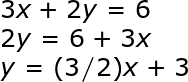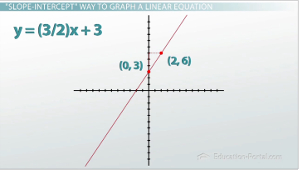# Linear Equations: Intercepts, Standard Form and Graphing

An error occurred trying to load this video.

Try refreshing the page, or contact customer support.

Coming up next: Abstract Algebraic Examples and Going from a Graph to a Rule

### You're on a roll. Keep up the good work!

Replay
Your next lesson will play in 10 seconds
• 0:06 How to Convert to…
• 1:52 'Slope-Intercept' Way…
• 3:48 'Standard Form' Way to…
• 5:41 Lesson Summary

Want to watch this again later?

Timeline
Autoplay
Autoplay
Speed

#### Recommended Lessons and Courses for You

Lesson Transcript
Instructor: Zach Pino
Do you know what to do if an equation doesn't look like y=mx+b?! If not, then this video is for you. Chances are the equation is in standard form, so we'll learn how to use standard form equations, how to graph them and why they can be helpful.

## How to Convert to Standard Form

This video is on the different forms of a linear equation, specifically slope-intercept form and standard form, and how we can graph lines given to us in either of those forms. Slope-intercept form (y = mx+b) is the one that most people are familiar with. It's the most common one you see but that doesn't mean it's the only way to represent a linear equation.

For example, if I had the line y = 3x+4, given to me in slope-intercept form, by using inverse operations and taking a positive 3x and undoing it with a -3x on both sides, I end up with the equation -3x+y = 4. These two equations are equivalent. They mean the same thing. They are the same thing, they're just written differently. What I end up with in this second one is what's called standard form. Essentially, because the xs and ys are on the same side of the equation. The generic standard form equation is Ax+By = C.This is probably the second most common form of a linear equation that you see, but unlike slope-intercept form, the As and Bs do not necessarily give us any useful information like the m and b does in the slope-intercept form. That doesn't mean that there aren't still some advantages to standard form over slope-intercept form.

## 'Slope-Intercept' Way to Graph a Linear Equation

What we're going to find out is that the orientation of the variables in standard form makes finding the x and y-intercepts pretty quick and easy, which will allow us to kind of use a shortcut when it comes to graphing.

Here we have a question that asks us to graph the line -3x+2y = 6. So let's do it the way we know how, which is by using slope-intercept form to use the m value and b value to graph our line. But because this isn't given to us in slope-intercept form, it requires us to first put it in slope-intercept form by using inverse operations to get the y by itself. This means we have to first undo the -3x with a positive 3x to both sides; we have to undo a times by 2 with a divide by 2 to both sides. Now we have the equation y = (3/2)x+3. This equation is equivalent to the one we started with, just written in a different way.Now that it's written in slope-intercept form, I know that I can use my m (my slope) and my b (my y-intercept) to graph it. I begin at 3 on the y-axis, and then I go up 3 and over 2 to find my next point using the slope. You could continue going up 3 and over 2 as many times as you wanted, but you notice that all your dots are in the same straight line. You can connect that line and you have your graph. Which isn't too bad, but these steps in the beginning that required us to first get the y by itself are unnecessary and sometimes they can be a little complicated, especially with the fractions. We'd rather know a way to do it without having to solve for y.To unlock this lesson you must be a Study.com Member.

### Register to view this lesson

Are you a student or a teacher?

### Unlock Your Education

#### See for yourself why 30 million people use Study.com

##### Become a Study.com member and start learning now.
Back
What teachers are saying about Study.com

### Earning College Credit

Did you know… We have over 160 college courses that prepare you to earn credit by exam that is accepted by over 1,500 colleges and universities. You can test out of the first two years of college and save thousands off your degree. Anyone can earn credit-by-exam regardless of age or education level.